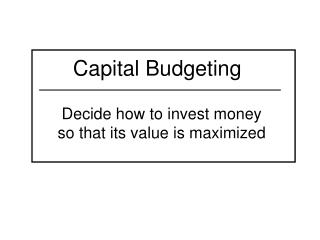DownloadDownload PresentationCapital Budgeting

# Capital Budgeting

Télécharger la présentation## Capital Budgeting

- - - - - - - - - - - - - - - - - - - - - - - - - - - E N D - - - - - - - - - - - - - - - - - - - - - - - - - - -
##### Presentation Transcript

1. Capital Budgeting Decide how to invest money so that its value is maximized

2. Capital Budgeting Process • Capital budget (investment) proposals are examined on basis of their cash outlays and resulting flow of future benefits over period of time greater than one year.

3. Capital Budgeting Process • Identify alternative investment opportunities and the capital required for each one. 2. Assess organizations ability to generate investment capital for capital budgeting period

4. Capital Budgeting Process 3. Measure cash (benefit) flows from alternative capital investment opportunities • Evaluate proposals using selected criteria Increase log inventory to reduce risk of mill downtime during Spring breakup?

5. Capital Budgeting Process • Select alternatives to fund and implement • Review performance for feed-backto decision makers Buy new skidder to reduce maintenance cost on old one and increase productivity?

6. Criteria to Rank Alternatives • Net Present Value • Internal Rate of Return • Benefit /Cost Ratio • Payback Period

7. Notation • ARR – alternative rate of return • B - annual nonmarket value, dollars • B/C - benefit/cost ratio • EAA - equivalent annual annuity • IRR - internal rate of return • MAR – minimum acceptable rate of return • N - project life, years • NPV = net present value

8. Notation • Cy – cost in year y • PV - present value • r - real interest rate • Ry - revenue in year y • y - index of years

9. Net Present Value NPV=∑ = ∑ n Ry Cy (1+r)y (1+r)y y=0 n Ry - Cy (1+r)y y=0

10. Example of NPV

11. Project D NPV C0 = - \$400/(1.06)0 = - \$ 400.00 C5 = - \$100/(1.06)5 = - \$ 74.73 R15 = \$200/(1.06)15 = \$ 83.45 R30 = \$6,600/(1.06)30 = \$1,149.13 NPV = 757.85

12. Comparison of PVC and PVR for example PV of revenues IRR PV of costs

13. Net Present Value Guideline • Project must at least cover the opportunity cost as measured by the minimum acceptable rate of return (MAR) used to calculate present values • Project is acceptable if NPV is zero or greater • Projects with negative NPV are unacceptable

14. Internal Rate of Return (IRR) • The r that makes NPV = 0 • Find by iterating over r until NPV = 0 • Meaning – r that makes PV of costs and PV of revenues equal

15. IRR Guideline • Project is acceptable if its IRR is equal to or greater than the minimum acceptable rate of return (MAR) • Relationship to NPV criteria – if MAR is the discount rate (r) used to calculate NPV, then IRR and NPV will accept same projects.

16. Benefit/Cost Ratio • PV (benefits)/PV (costs), or • PV (revenues)/PV (expenses) ∑ Ry/(1+r)y = ∑ Cy/(1+r)y n n y=0 n y=0

17. Benefit/Cost Ratio Guideline • Accept project if B/C ≥ 1.0 • If B/C ≥ 1.0 then • NPV ≥ 0, and • IRR ≥ MAR

18. Relationship of NPV, IRR and B/C IRR B/C > 1 NPV = 0 B/C < 1 NPV < 0 PV of revenues PV of costs

19. Payback Period • Time required for net revenue to equal invested capital • Example, • Invest \$10,000 • Net revenue is \$5,000 per year • Payback is 2 years, (\$10,000/\$5,000) • Best used in conjunction with other criteria

20. Ranking Projects • NPV, IRR, and B/C may not rank alternative projects in the same order • Additional ranking criteria • Mutually exclusive projects – only one can be chosen • Independent • Opposite of mutually exclusive, • Can all be adopted

21. Ranking Projects • Additional ranking criteria, cont. • Divisible – can invest in part of a project • Indivisible – all or nothing

22. Example of NPV

23. Timing of cash flows affects rankings • Alternatives D and N have same total cost and revenue • Rankings by NPV and IRR are different depending on MAR

24. NPV NPV project D NPV same at 6.3% NPV project N IRR=14.5% IRR = 9.7% Interest Rate 2 4 6 10 14